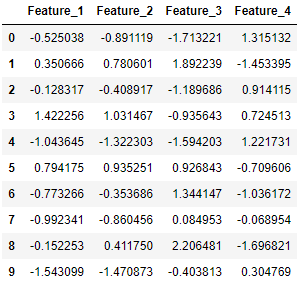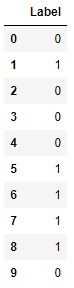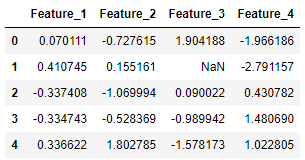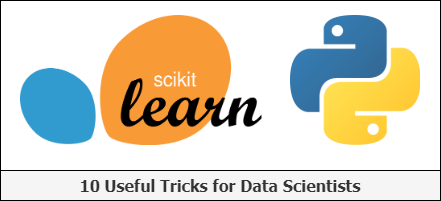1595414820

# Scikit-Learn (Python): 6 Useful Tricks for Data Scientists

**Scikit-learn (sklearn) **is a powerful open source **machine learning library **built on top of the Python programming language. This library contains a lot of efficient tools for machine learning and statistical modeling, including various classification, regression, and clustering algorithms.

In this article, I will show 6 tricks regarding the scikit-learn library to make certain programming practices a bit easier.

# 1. Generate random dummy data

To generate random ‘dummy’ data, we can make use of the `make_classification()` function in case of classification data, and `make_regression()` function in case of regression data. This is very useful in some cases when debugging or when you want to try out certain things on a (small) random data set.

Below, we generate 10 classification data points consisting of 4 features (found in X) and a class label (found in y), where the data points belong to either the negative class (0) or the positive class (1):

``````from sklearn.datasets import make_classification
import pandas as pd

X, y = make_classification(n_samples=10, n_features=4, n_classes=2, random_state=123)
``````

Here, X consists of the 4 feature columns for the generated data points:

``````pd.DataFrame(X, columns=['Feature_1', 'Feature_2', 'Feature_3', 'Feature_4'])
``````And y contains the corresponding label of each data point:

``````pd.DataFrame(y, columns=['Label'])
``````# 2. Impute missing values

Scikit-learn offers multiple ways to impute missing values. Here, we consider two approaches. The `SimpleImputer` class provides basic strategies for imputing missing values (through the mean or median for example). A more sophisticated approach the `KNNImputer` class, which provides imputation for filling in missing values using the K-Nearest Neighbors approach. Each missing value is imputed using values from the `n_neighbors` nearest neighbors that have a value for the particular feature. The values of the neighbors are averaged uniformly or weighted by distance to each neighbor.

Below, we show an example application using both imputation methods:

``````from sklearn.experimental import enable_iterative_imputer
from sklearn.impute import SimpleImputer, KNNImputer
from sklearn.datasets import make_classification
import pandas as pd
X, y = make_classification(n_samples=5, n_features=4, n_classes=2, random_state=123)
X = pd.DataFrame(X, columns=['Feature_1', 'Feature_2', 'Feature_3', 'Feature_4'])
print(X.iloc[1,2])
``````

2.21298305

Transform X[1, 2] to a missing value:

``````X.iloc[1, 2] = float('NaN')

X
``````First we make use of the simple imputer:

``````imputer_simple = SimpleImputer()

pd.DataFrame(imputer_simple.fit_transform(X))
``````

#machine-learning #data-science #scikit-learn #programming #python #deep learning

## Buddha Community1619518440

## top 30 Python Tips and Tricks for Beginners

Welcome to my Blog , In this article, you are going to learn the top 10 python tips and tricks.

### 8) Check The Memory Usage Of An Object.

#python #python hacks tricks #python learning tips #python programming tricks #python tips #python tips and tricks #python tips and tricks advanced #python tips and tricks for beginners #python tips tricks and techniques #python tutorial #tips and tricks in python #tips to learn python #top 30 python tips and tricks for beginners1595414820

## Scikit-Learn (Python): 6 Useful Tricks for Data Scientists

**Scikit-learn (sklearn) **is a powerful open source **machine learning library **built on top of the Python programming language. This library contains a lot of efficient tools for machine learning and statistical modeling, including various classification, regression, and clustering algorithms.

In this article, I will show 6 tricks regarding the scikit-learn library to make certain programming practices a bit easier.

# 1. Generate random dummy data

To generate random ‘dummy’ data, we can make use of the `make_classification()` function in case of classification data, and `make_regression()` function in case of regression data. This is very useful in some cases when debugging or when you want to try out certain things on a (small) random data set.

Below, we generate 10 classification data points consisting of 4 features (found in X) and a class label (found in y), where the data points belong to either the negative class (0) or the positive class (1):

``````from sklearn.datasets import make_classification
import pandas as pd

X, y = make_classification(n_samples=10, n_features=4, n_classes=2, random_state=123)
``````

Here, X consists of the 4 feature columns for the generated data points:

``````pd.DataFrame(X, columns=['Feature_1', 'Feature_2', 'Feature_3', 'Feature_4'])
``````And y contains the corresponding label of each data point:

``````pd.DataFrame(y, columns=['Label'])
``````# 2. Impute missing values

Scikit-learn offers multiple ways to impute missing values. Here, we consider two approaches. The `SimpleImputer` class provides basic strategies for imputing missing values (through the mean or median for example). A more sophisticated approach the `KNNImputer` class, which provides imputation for filling in missing values using the K-Nearest Neighbors approach. Each missing value is imputed using values from the `n_neighbors` nearest neighbors that have a value for the particular feature. The values of the neighbors are averaged uniformly or weighted by distance to each neighbor.

Below, we show an example application using both imputation methods:

``````from sklearn.experimental import enable_iterative_imputer
from sklearn.impute import SimpleImputer, KNNImputer
from sklearn.datasets import make_classification
import pandas as pd
X, y = make_classification(n_samples=5, n_features=4, n_classes=2, random_state=123)
X = pd.DataFrame(X, columns=['Feature_1', 'Feature_2', 'Feature_3', 'Feature_4'])
print(X.iloc[1,2])
``````

2.21298305

Transform X[1, 2] to a missing value:

``````X.iloc[1, 2] = float('NaN')

X
``````First we make use of the simple imputer:

``````imputer_simple = SimpleImputer()

pd.DataFrame(imputer_simple.fit_transform(X))
``````

#machine-learning #data-science #scikit-learn #programming #python #deep learning1625843760

## Python Packages in SQL Server – Get Started with SQL Server Machine Learning Services

##### Introduction

When installing Machine Learning Services in SQL Server by default few Python Packages are installed. In this article, we will have a look on how to get those installed python package information.

##### Python Packages

When we choose Python as Machine Learning Service during installation, the following packages are installed in SQL Server,

• revoscalepy – This Microsoft Python package is used for remote compute contexts, streaming, parallel execution of rx functions for data import and transformation, modeling, visualization, and analysis.
• microsoftml – This is another Microsoft Python package which adds machine learning algorithms in Python.
• Anaconda 4.2 – Anaconda is an opensource Python package

#machine learning #sql server #executing python in sql server #machine learning using python #machine learning with sql server #ml in sql server using python #python in sql server ml #python packages #python packages for machine learning services #sql server machine learning services1620466520

If you accumulate data on which you base your decision-making as an organization, you should probably think about your data architecture and possible best practices.

If you accumulate data on which you base your decision-making as an organization, you most probably need to think about your data architecture and consider possible best practices. Gaining a competitive edge, remaining customer-centric to the greatest extent possible, and streamlining processes to get on-the-button outcomes can all be traced back to an organization’s capacity to build a future-ready data architecture.

In what follows, we offer a short overview of the overarching capabilities of data architecture. These include user-centricity, elasticity, robustness, and the capacity to ensure the seamless flow of data at all times. Added to these are automation enablement, plus security and data governance considerations. These points from our checklist for what we perceive to be an anticipatory analytics ecosystem.

#big data #data science #big data analytics #data analysis #data architecture #data transformation #data platform #data strategy #cloud data platform #data acquisition1593156510

## Basic Data Types in Python | Python Web Development For Beginners

At the end of 2019, Python is one of the fastest-growing programming languages. More than 10% of developers have opted for Python development.

In the programming world, Data types play an important role. Each Variable is stored in different data types and responsible for various functions. Python had two different objects, and They are mutable and immutable objects.

I Mutable objects

II Immutable objects

III Built-in data types in Python

## Mutable objects

The Size and declared value and its sequence of the object can able to be modified called mutable objects.

Mutable Data Types are list, dict, set, byte array

## Immutable objects

The Size and declared value and its sequence of the object can able to be modified.

Immutable data types are int, float, complex, String, tuples, bytes, and frozen sets.

id() and type() is used to know the Identity and data type of the object

a**=25+**85j

type**(a)**

output**:<class’complex’>**

b**={1:10,2:“Pinky”****}**

id**(b)**

output**:**238989244168

## Built-in data types in Python

a**=str(“Hello python world”)****#str**

b**=int(18)****#int**

c**=float(20482.5)****#float**

d**=complex(5+85j)****#complex**

e**=list((“python”,“fast”,“growing”,“in”,2018))****#list**

f**=tuple((“python”,“easy”,“learning”))****#tuple**

g**=range(10)****#range**

h**=dict(name=“Vidu”,age=36)****#dict**

i**=set((“python”,“fast”,“growing”,“in”,2018))****#set**

j**=frozenset((“python”,“fast”,“growing”,“in”,2018))****#frozenset**

k**=bool(18)****#bool**

l**=bytes(8)****#bytes**

m**=bytearray(8)****#bytearray**

n**=memoryview(bytes(18))****#memoryview**

## Numbers (int,Float,Complex)

Numbers are stored in numeric Types. when a number is assigned to a variable, Python creates Number objects.

#signed interger

age**=**18

print**(age)**

Output**:**18

Python supports 3 types of numeric data.

int (signed integers like 20, 2, 225, etc.)

float (float is used to store floating-point numbers like 9.8, 3.1444, 89.52, etc.)

complex (complex numbers like 8.94j, 4.0 + 7.3j, etc.)

A complex number contains an ordered pair, i.e., a + ib where a and b denote the real and imaginary parts respectively).

## String

The string can be represented as the sequence of characters in the quotation marks. In python, to define strings we can use single, double, or triple quotes.

# String Handling

‘Hello Python’

#single (') Quoted String

“Hello Python”

# Double (") Quoted String

“”“Hello Python”“”

‘’‘Hello Python’‘’

# triple (‘’') (“”") Quoted String

In python, string handling is a straightforward task, and python provides various built-in functions and operators for representing strings.

The operator “+” is used to concatenate strings and “*” is used to repeat the string.

“Hello”+“python”

output**:****‘Hello python’**

"python "*****2

'Output : Python python ’

#python web development #data types in python #list of all python data types #python data types #python datatypes #python types #python variable type